Courses
Courses for Kids
Free study material
Free LIVE classes
More

# Understanding Division with Real-Life Examples!## What is Division?

In general, kids begin to learn division in-depth in the third grade; however, in the first and second years, kids will be introduced to concepts that lead to long-form division and simple division using methods and games. Kids are expected to work with numbers far greater than those they can physically count as mathematical concepts become more complex. Understanding division will be easier when learned with real-life examples. Let's explore division for year 2 kids in this article.

## What is the Definition of Division?

The division is a process of repeated subtraction. It is the inverse action of multiplication. It is considered the formation of equal groups. When we divide numbers, we split them down into smaller numbers so that the multiplication of those smaller numbers equals the larger number taken.

## Daily Life Examples

1. Nirmal has 12 Sweets and he wants to share them equally between his three friends. Let's start by creating three groups then add one sweet at a time into each group until all twelve sweets have been shared equally. Let's count how many sweets are in each group to get an answer: Twelve sweets shared equally between friends is 4.

The division is denoted by a mathematical symbol that consists of a small horizontal line with a dot each above and below the line. There are two basic division symbols that represent the division of two numbers.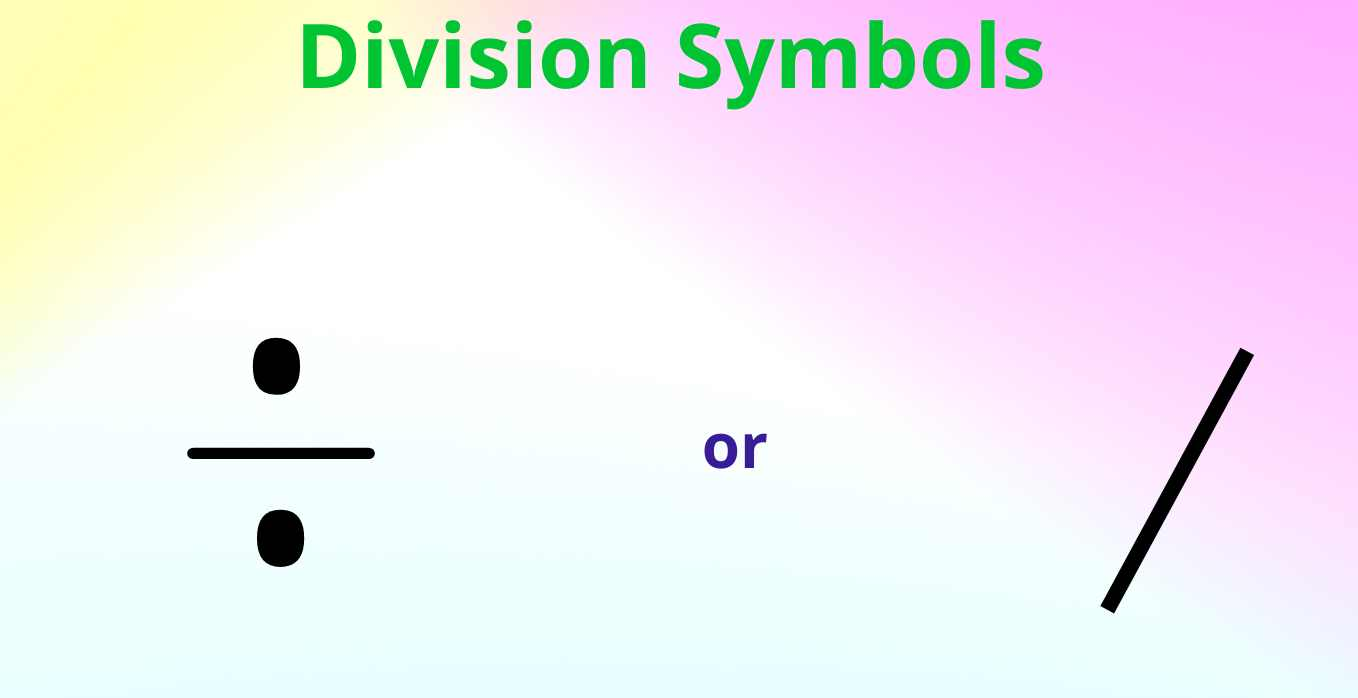Symbols for Division

Other words for division are ‘share between’ and ‘divided’. Our solved example can be written as: 12 divided by 3 equals 4.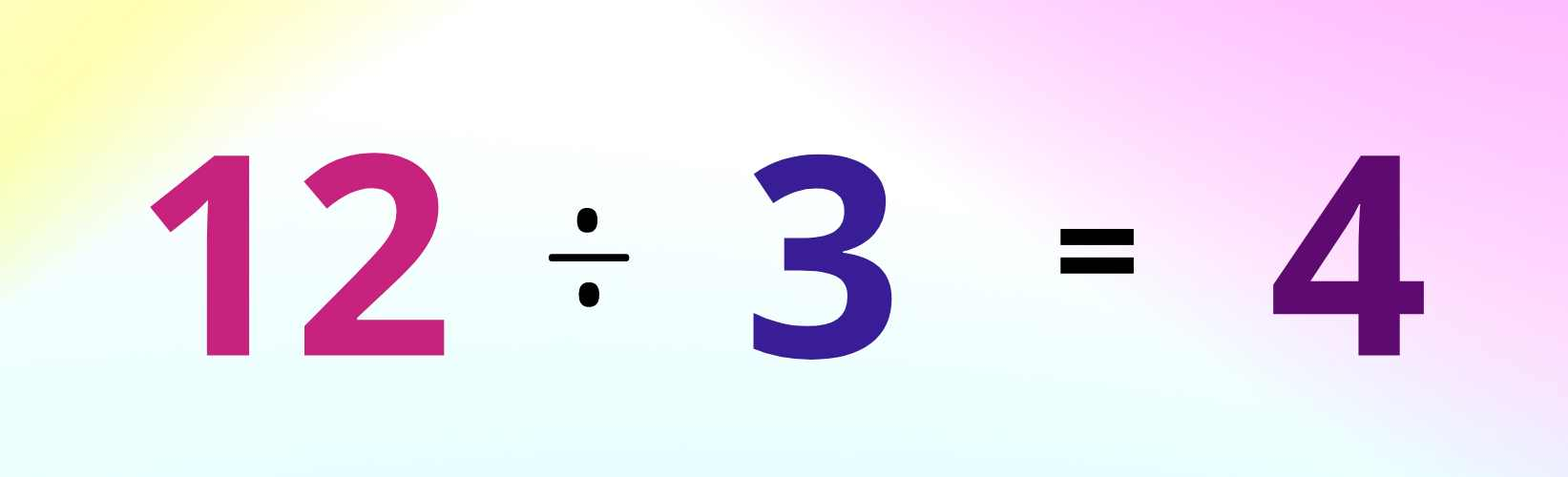Image Shows the Division 12/3

2. Mahima has 16 apples and wants to divide them equally between her 4 friends. This can be written as 16 divided by 4.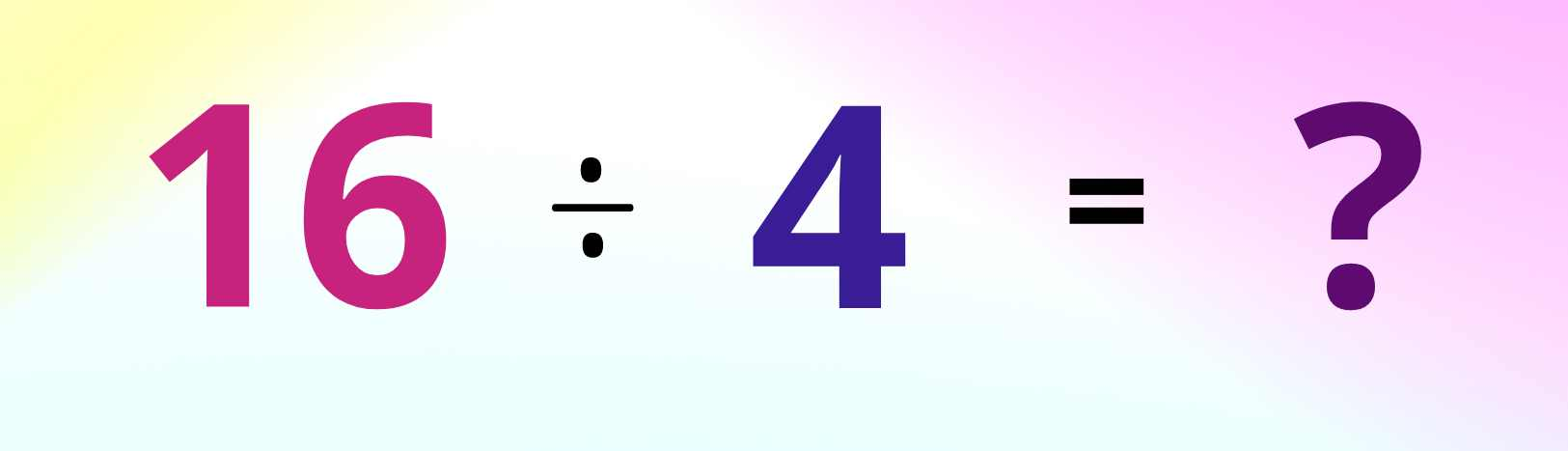Image Shows the Division 16/4

To find the answer we need to share 16 equally between 4 groups. Let's count how many apples are in each group to get our answer.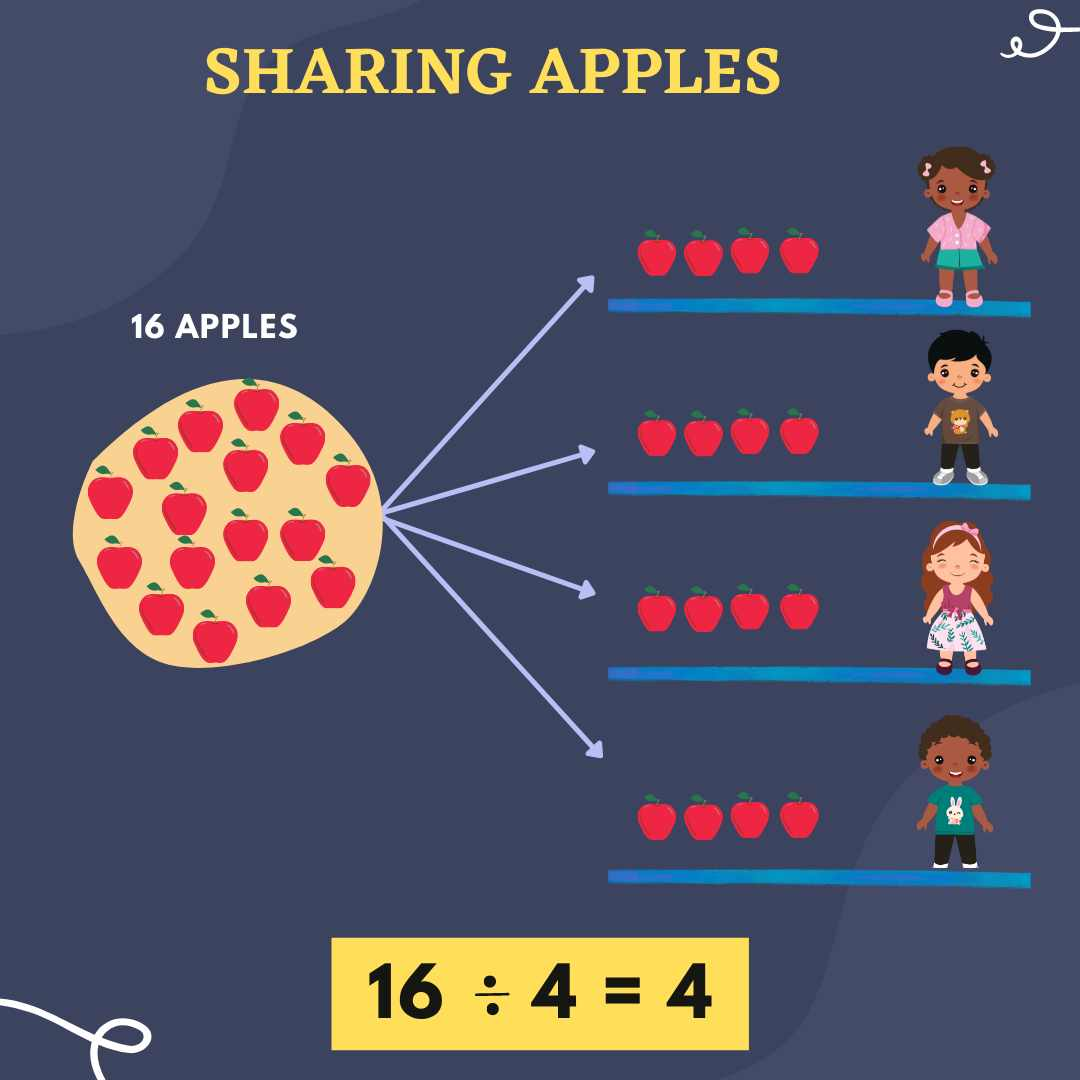The Image Shows 16 Apples Shared Equally Among 4 People With the Division of 16/4

So, 16 apples shared equally between friends is 4.

3. We can divide by sharing objects into equal groups. So if we make the calculation, for 10 divided by 2, we can work out the answer by sharing ten objects equally into two groups.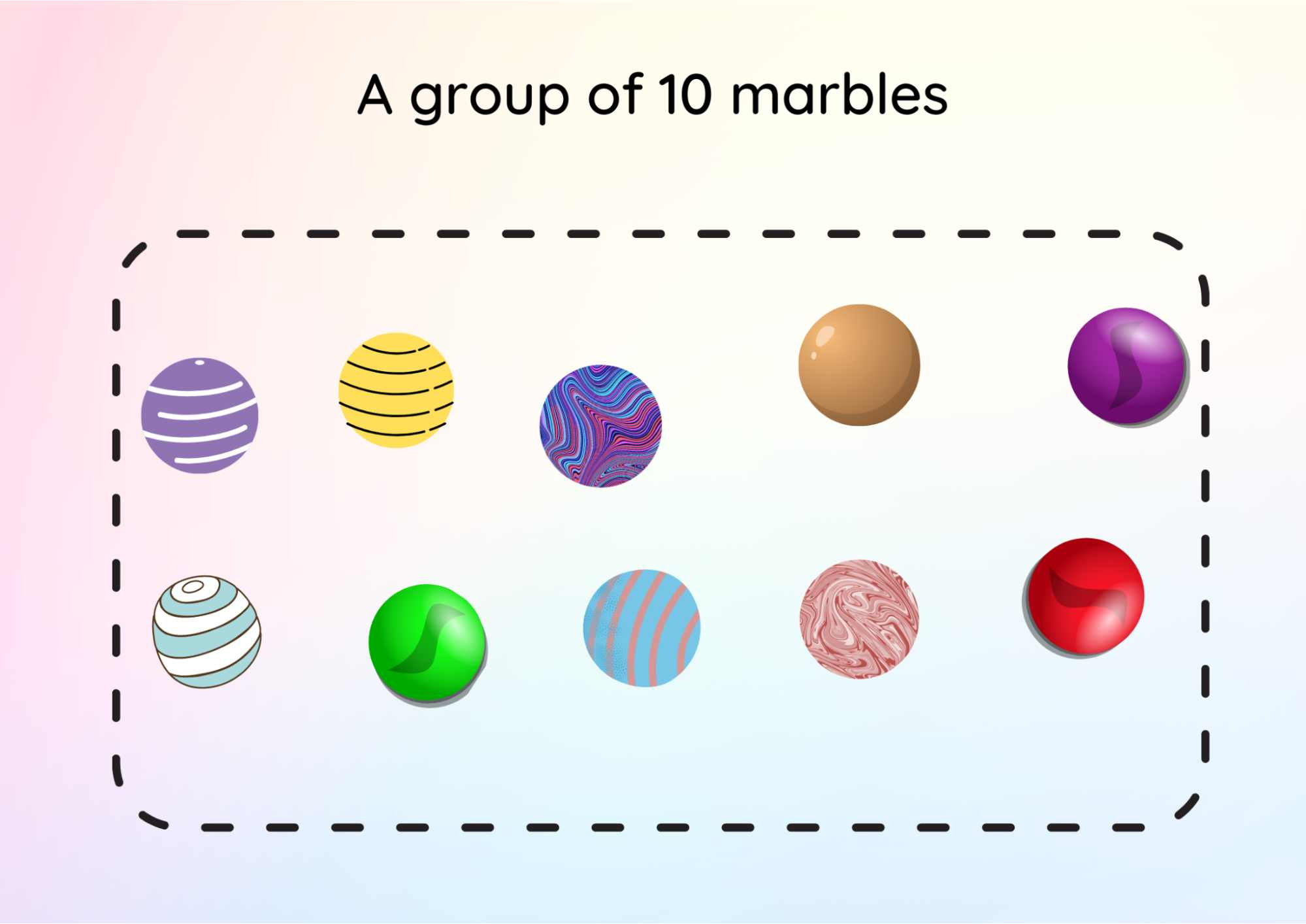The Image Shows a Group of 10 Objects

There are five marbles in each of the two groups.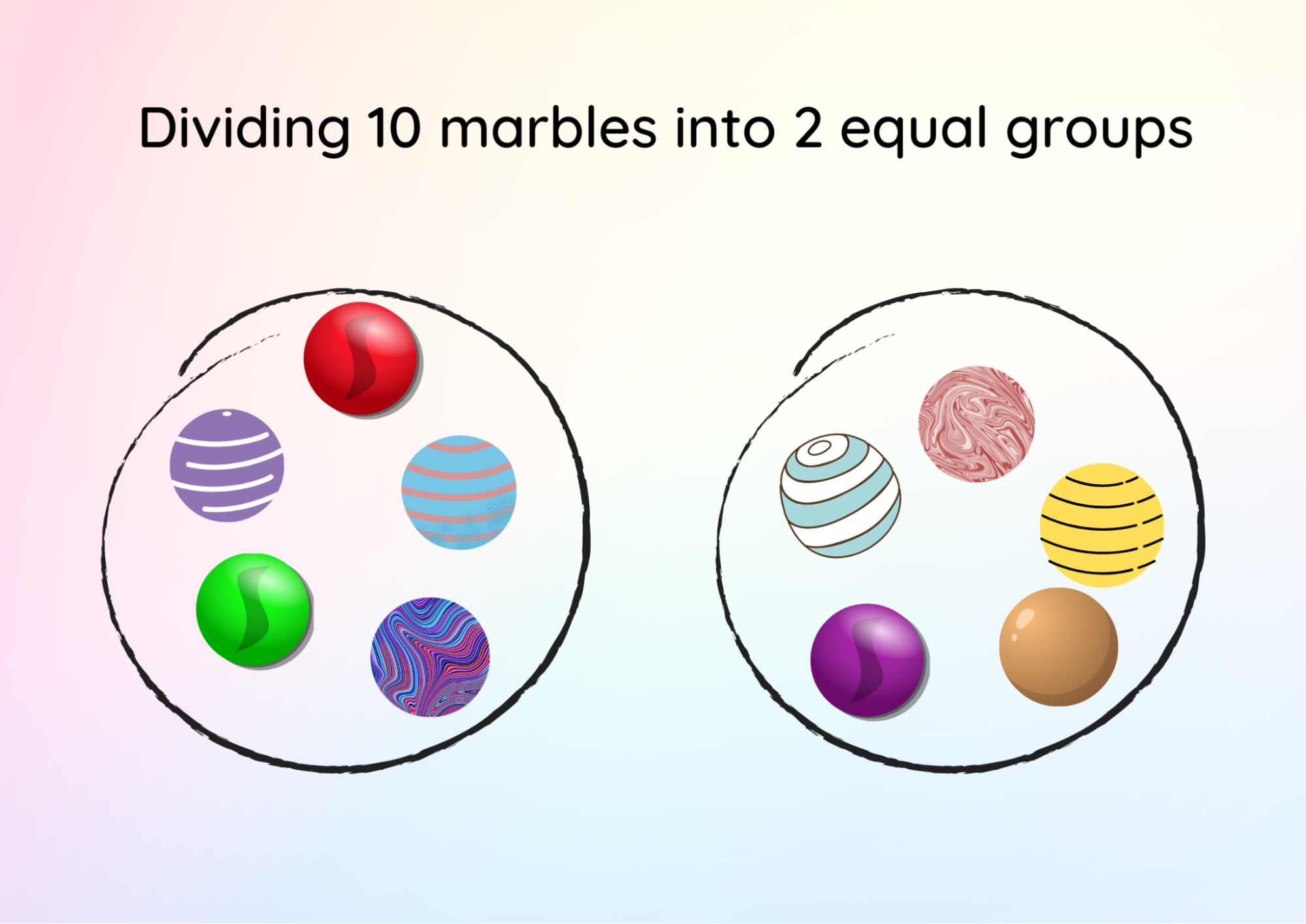Image Shows 10 Objects Which are Equally Divided Into 2 Groups Each of 5 Objects

So, ten divided by two equals five.

## Division by Grouping

Division by grouping looks at how many equal groups there are in a number. So if we take the calculation same as story example 3,

10 divided by 2.

We can look at this as how many groups of 2 there are in 10.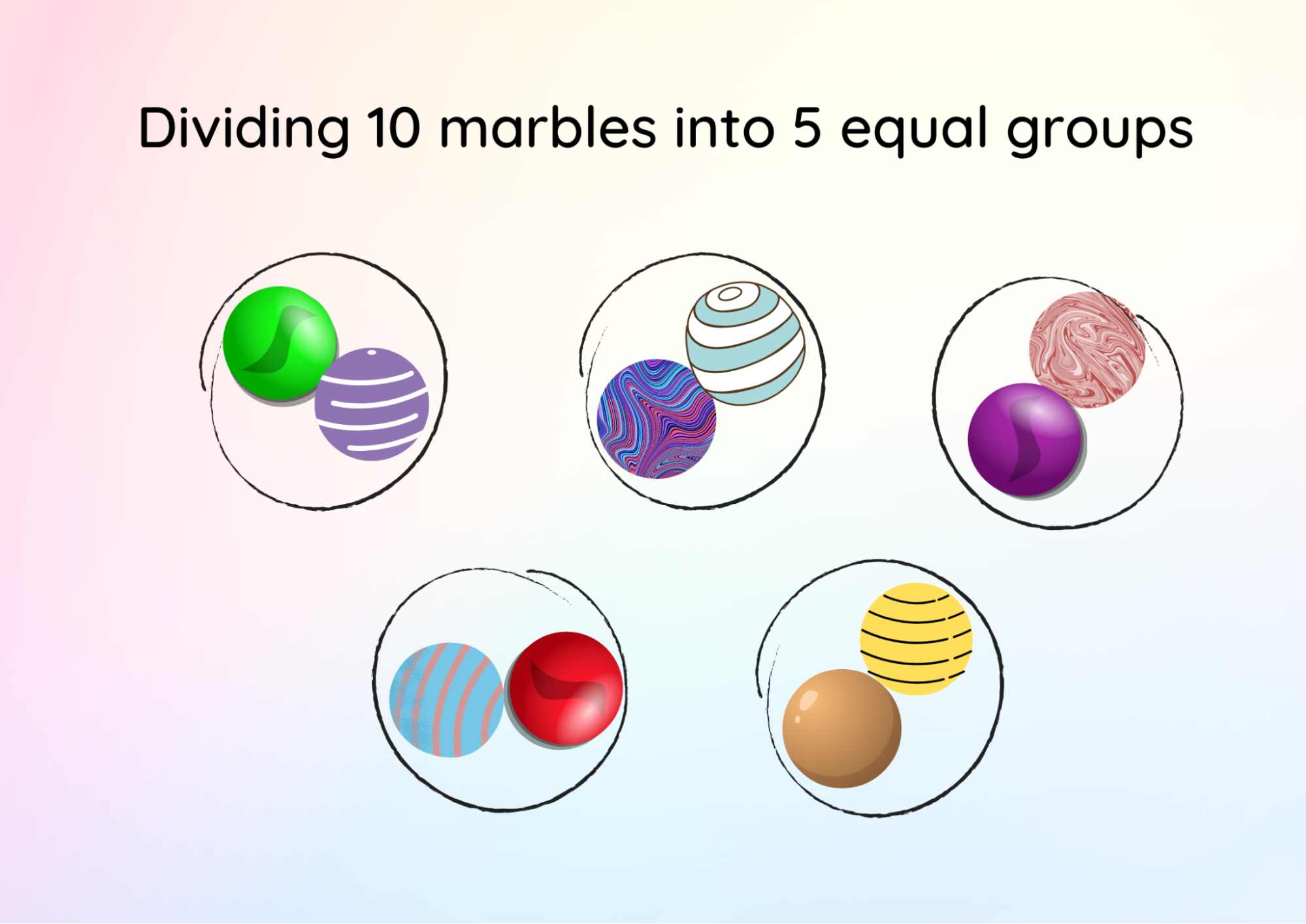Image Shows 10 Objects Which are Equally Divided Into 5 Groups Each of 2 Objects

Equal groups of 2 in 10 are 5 (Refer to the image above). So 10 divided by 2 equals 5.

## Important Terms in Division

• The number to be divided in a division sum is referred to as the dividend.

• The divisor is the number by which we divide.

• The result of division is known as the quotient.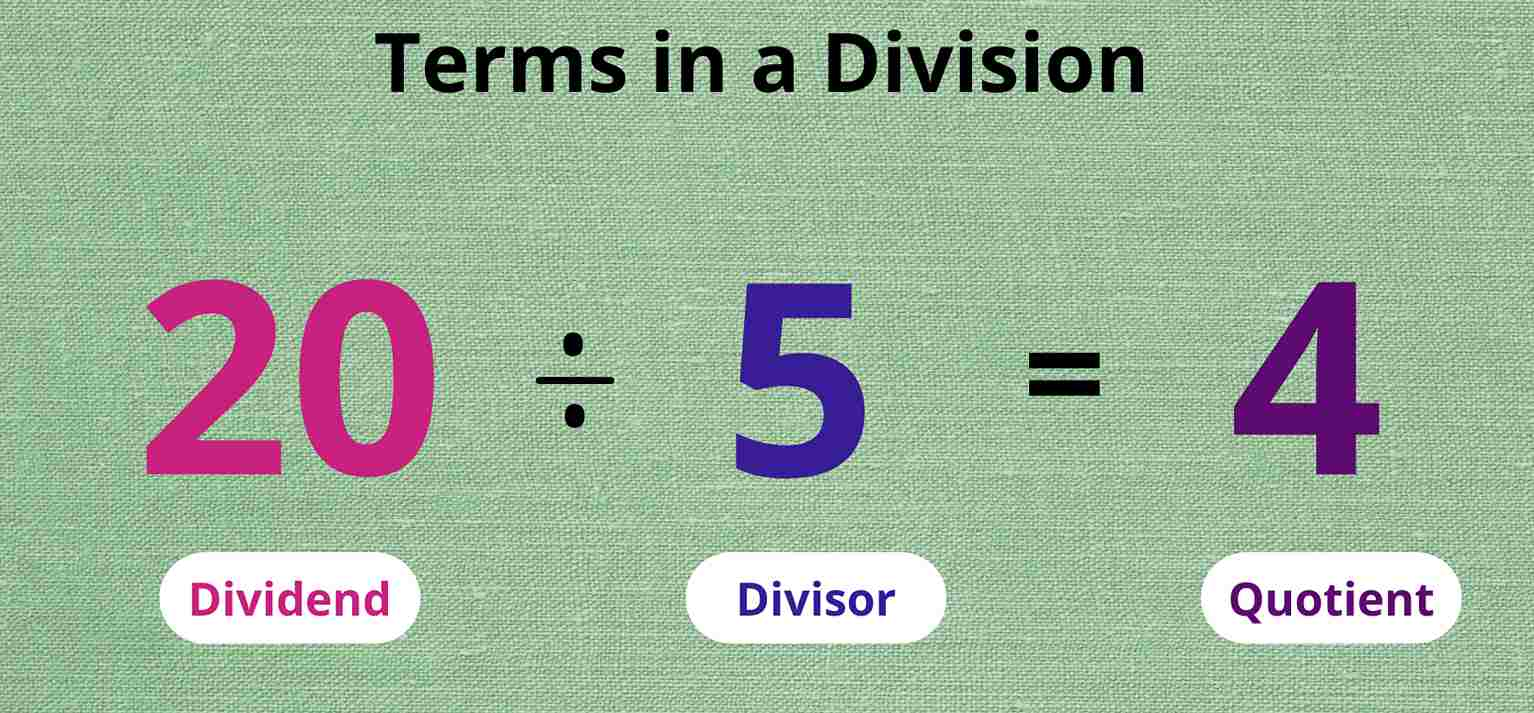Image Shows the Terms of a Division with an Example

## Multiplication and Division

The below picture shows 12 candies arranged in groups of 4.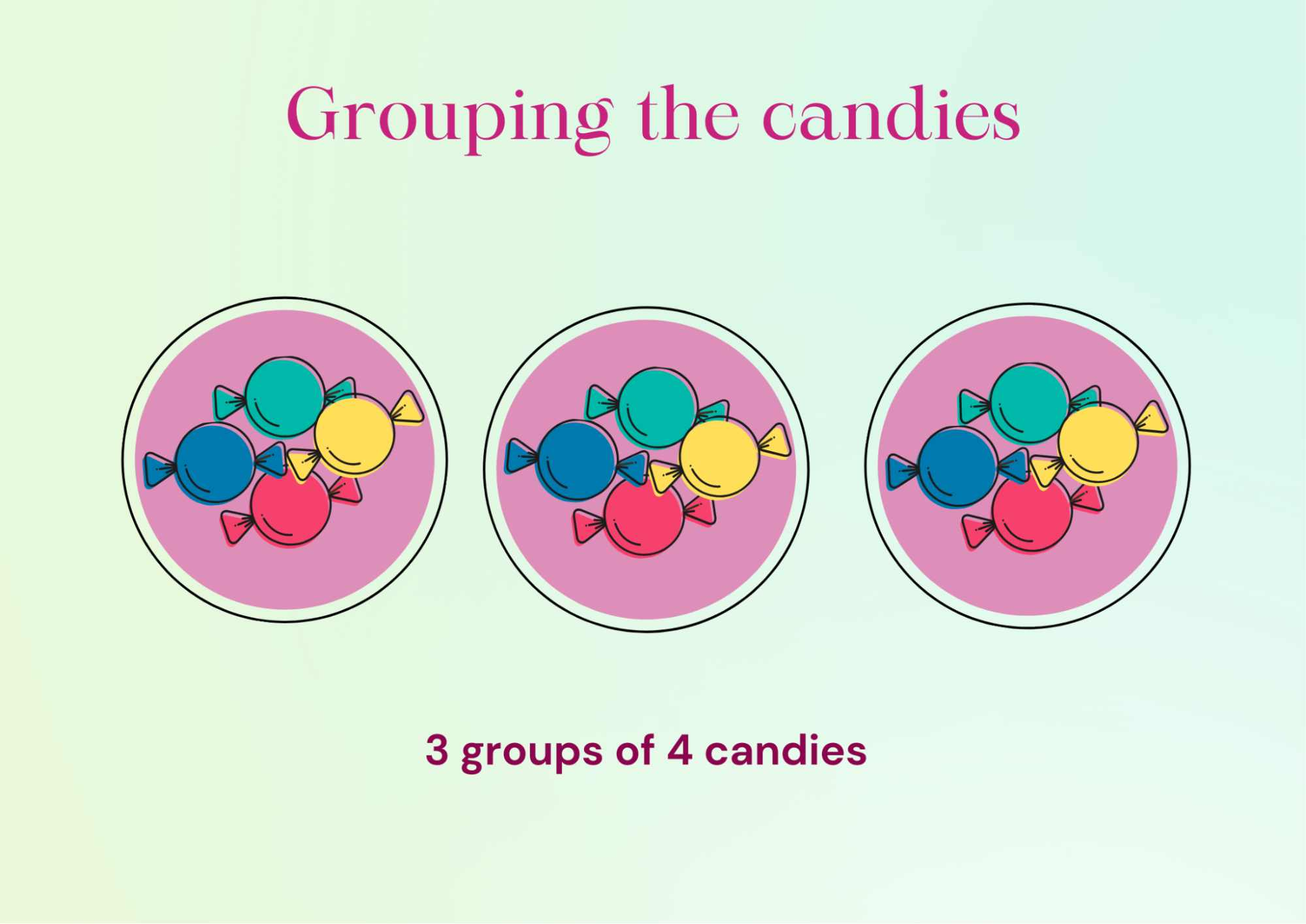Image Shows Three Groups Each of Four Candies

If we observe, it shows a multiplication fact: 3 x 4 = 12 and also a division fact: 12 ÷ 4 = 3. 12 candies can be arranged in groups of 3 as well. It is shown below.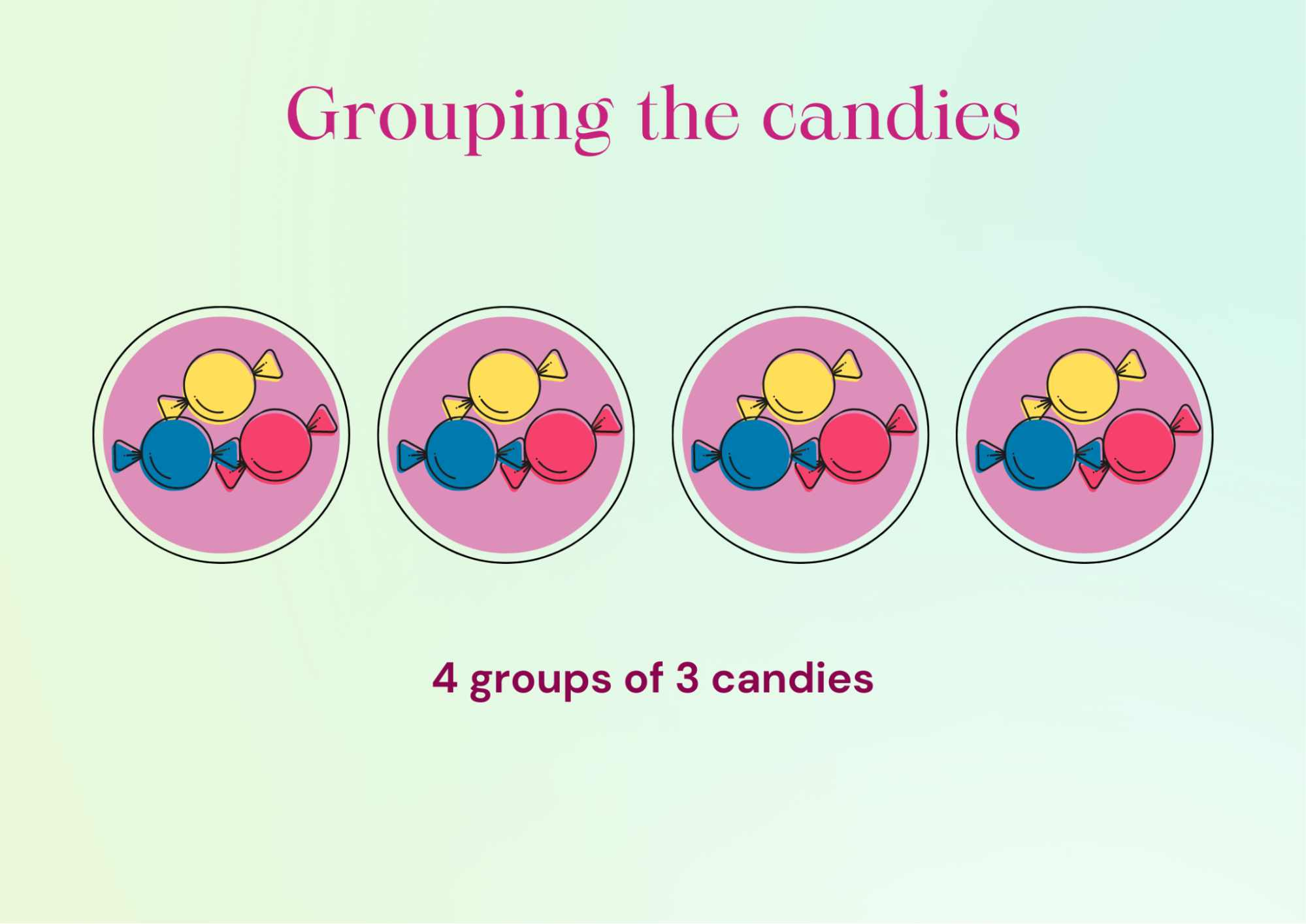The Image Shows Four Groups Each of Three Candies

Here, we have a multiplication fact: 4 x 3 = 12 and a division fact: 12 ÷ 3 = 4.

Thus, we can observe that,

• Multiplication of 3 x 4 = 12 gives us the division fact 12 ÷ 4 = 3.

• Multiplication of 4 x 3 = 12 gives us the division fact 12 ÷ 3 = 4.

## Helping Kids with Division at Home

Toys, some grapes, paper clips and buttons are all terrific practice items. Ask your child to count the number of objects, to begin with. Can they distribute the items equally between the two of you? Assist them in sharing objects between the two groups one at a time. When all of the objects have been divided, ask your child how many of each group there are. If you have the same number, explain that your child divided the objects equally among two groups, which is equal to dividing by two.

## Conclusion

The division is the splitting of a large group into smaller groups so that each group has an equal number of things. In mathematics, it is an operation used for equal grouping and equal sharing. In this article, we have discussed the division process in maths in detail with some examples.

Last updated date: 28th Sep 2023
Total views: 122.4k
Views today: 3.22k

## FAQs on Understanding Division with Real-Life Examples!

1. What are the 3 parts of division?

Dividend, divisor, and quotient are the three basic parts of every division process. The remainder is another element that is either 0 in certain problems or an integer in others.

2. What is short division?

Short division is a quick and efficient way to solve division problems with greater numbers. Short division can be used to solve division problems with very big dividends by following a series of simple steps.

3. What is long division in maths?

Long division is a method for dividing a large number by a two-digit (or greater) number. It is written out in a similar approach, which is used for short division.